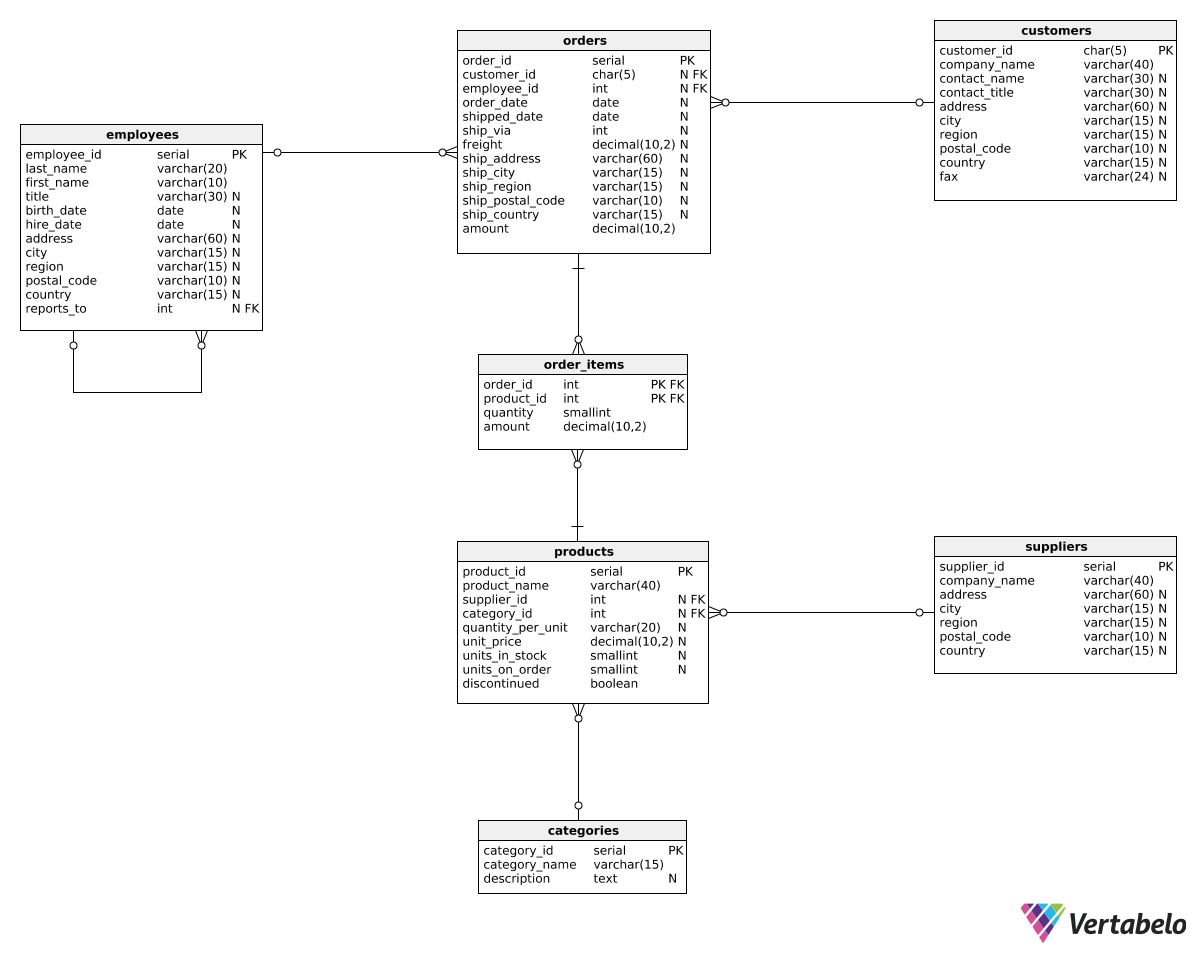Deals Of The Week - hours only!Up to 80% off on all courses and bundles.-Close
Introduction
Reports with revenue in categories
Revenue in time periods for selected categories
Summary

## Instruction

Good job! Now that we know how to add total_revenue as the last column, we can calculate the ratio between a category's revenue and total revenue. Such a report can help us quickly identify which categories generate the most revenue. Here's the query:

WITH all_categories AS (
SELECT SUM(amount) AS total_sum
FROM orders
)
SELECT
category_name,
SUM(oi.amount) AS category_revenue,
SUM(oi.amount) / CAST(ac.total_sum AS decimal(10,2)) AS total_revenue_ratio
FROM all_categories ac, order_items oi
JOIN orders o
ON o.order_id = oi.order_id
JOIN products p
ON oi.product_id = p.product_id
JOIN categories c
ON p.category_id = c.category_id
GROUP BY category_name, ac.total_sum;


In the third column, we now divide category revenue by total revenue and get the correct ratio. As usual, we're casting one of the values to a decimal type to avoid integer division.

## Exercise

Create a report of the category revenue for all orders placed in 2017 or later. Show three columns: category_name, category_revenue, and total_revenue_percentage. The last column should show the rounded to two decimal places percentage of the total revenue generated by that category.

### Stuck? Here's a hint!

Filter rows in both the CTE and the outer query. To get percentages, multiply the ratio of category revenue to total revenue by 100.